# One-Variable Data Table In ExcelArticle byExcelMojo Team## What Is One-Variable Data Table In Excel?

The One-Variable Data Table in Excel is a generated data table using the What-if analysis. It shows the impact of one input variable provided as a row or a column value on a formula outcome. And the specific formula in a one-variable data table should refer to a single input cell.

Users can use the one-variable data table to determine monthly payments for different interest rates during financial analysis.

For example, the below image shows two tables. The first table contains the dimensions of a rectangle in meters, with the rectangle volume calculated in cell B7 using the given dimensions.

We will create a One-Variable Data Table to see how the rectangle length influences the volume and display the output in the second table.

Select cell range, D3:E7, and select DataWhat-If AnalysisData Table to open the Data Table window. And once we enter the column input cell in the Data Table window, as the given input variable values are in a single column, we will get the required volume values in cells E4:E7.

We get the generated One-Variable Data Table in Excel, as shown above.

[Output Observation: Enter the formula in cell B7 to get the required rectangle volumes, and also cell E3 should have the formula reference. And then, we will get the excel data table in cell range E4:E7, the length, breadth, and height values will be 50 (cell D7), 20, and 30 meters. And thus, the calculated rectangle volume is 30000 m3.]

###### Key Takeaways
• The One-Variable Data Table in Excel helps evaluate a formula output based on the changes made to the value of a single input variable.
• Users can use the One-Variable Data Table to determine the best interest rate for paying a possible monthly EMI or loan amount.
• To build a One-Variable Data Table, first, enter the required equation value, with the single input variable, in the first cell of the target cell range. And the first row or column of the target cell range will have the input variable values. Accordingly, we should select the target cell range and choose DataWhat-If AnalysisData Table to enter the row or column input cell reference. It will result in the required one-variable data table.

### How To Create One-Variable Data Table In Excel?

The steps to build a One-Variable Data Table are as follows:

• First, ensure the formula and the input variable values, based on which we need to build the one-variable data table, are accurate.
• Then, select the target cell range, choose DataWhat-If AnalysisData Table, and enter the row or column input for creating a One-Variable Data Table in Excel.
• Finally, press OK in the Data Table window to view the resulting one-variable data table based on the given single input variable values.

We will create One-Variable Data Table with the following example.

The below image shows two tables. The first table contains the required parameters to compute the compound interest, and it shows the calculated balance in cell B9, having the data format Currency.

The steps to create One-Variable Data Table in Excel are as follows:

1. Ensure cell E3 has the cell reference to the formula in cell B9.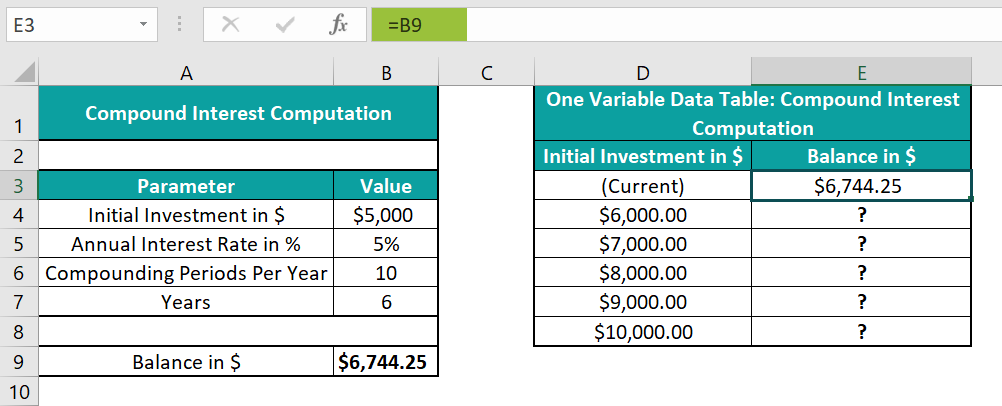2. Choose cell range, D3:E8 → select the “Data” tab → go to the “Forecast” group → click the “What-If Analysis” option drop-down → select the “Data Table” option, as shown below.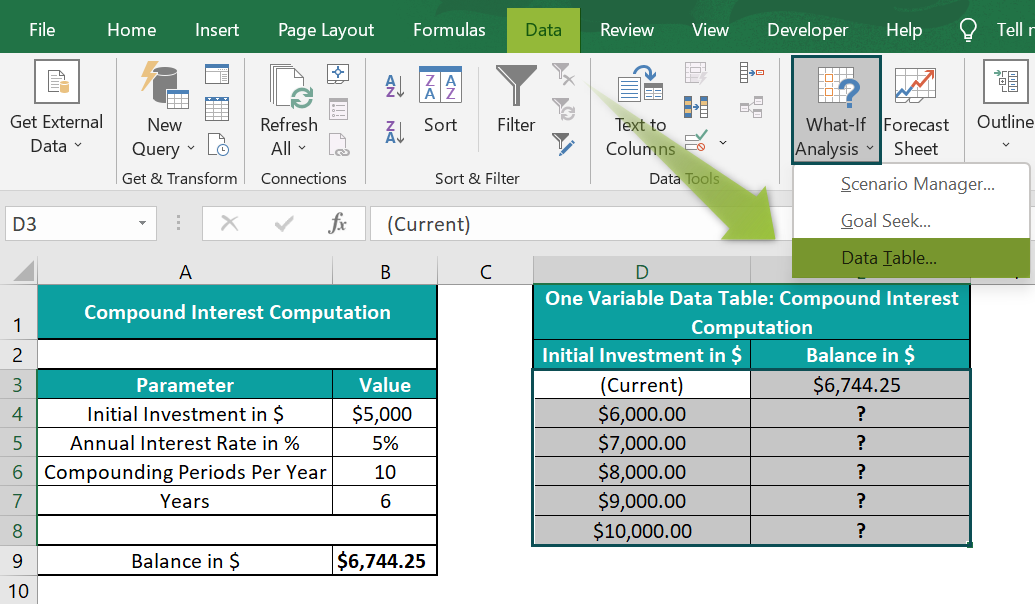3. In the “Data Table” dialog box that pops up, enter the cell reference to the initial investment value in the first table, cell B4, in the “Column input cell:” field [since we have the column range selected to get the generated data table], as shown below.

[Note: However, if the input variable, Initial Investment, values were in a row range. Then we would require to enter the “Row input cell:” value in the Data Table window, which will be the cell reference to the initial investment value in the first table, cell B4.]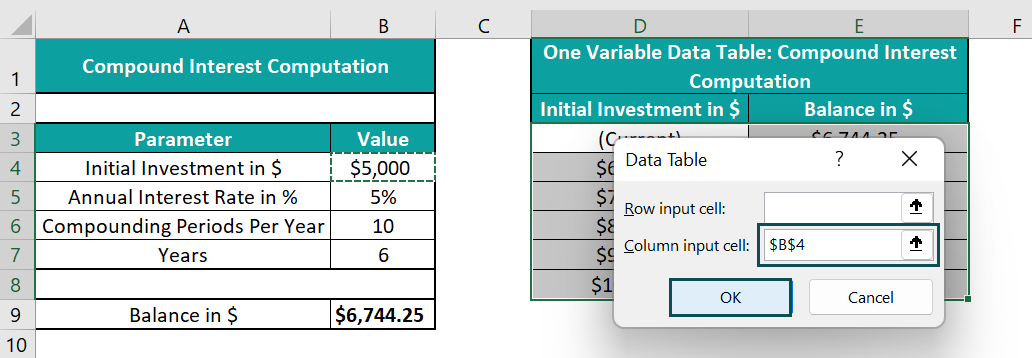4. Click “OK” in the Data Table window, and set the data format of cell E4:E8 as Currency.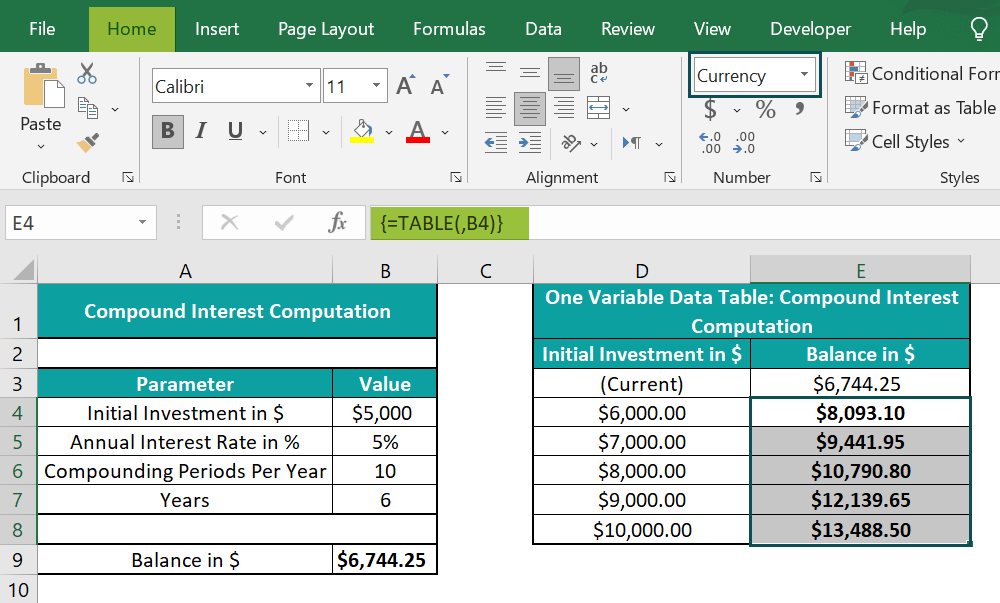We get the generated One-Variable Data Table, as shown above.

[Output Observation: Let us consider the cell E8 value. The compound index formula takes the initial investment value as \$10,000.00 (cell D8 value), with the remaining parameters taking the values from the first table. And based on the given initial investment value, the compound interest gets computed as \$13,488.50 in cell E8. Hence, the initial investment variable impacts the compound interest values.]

### Examples

We will understand some advanced scenarios with the One-Variable Data Table in Excel examples.

#### Example #1

We will create a One-Variable Data Table in Excel, where a single input variable impacts the outcome of multiple formulas.

The below image shows two tables. The first table contains the input parameters required to perform the loan payment computation.

The steps to create a One-Variable Data Table for the loan payment computation in the second table are,

• Step 1: First, select cell B11, and enter the formula =B4*(1-B5) to determine the loan amount.

Then, select cell B12, and enter the formula=PMT(B7/12,B6,-B11) to determine the monthly payment.

Next, select cell B13, and enter the formula =B12*B6 to determine the total payment.

Finally, select cell B14, and enter the formula=B13-B11 to determine the total interest.

• Step 2: We shall start building the one-variable data table by entering the formula for each output parameter in columns F:I.

Then, select cell F3, and provide Excel cell reference to the B11 cell’s formula.

Next, we must select cell G3, and provide cell reference to the B12 cell’s formula.

Then, select cell H3, and provide cell reference to the B13 cell’s formula.

Finally, select cell I3, and provide cell reference to the B14 cell’s formula.

• Step 3: We now have the required interest rates and the formulas that they affect. So, here is how we can create the required one-variable data table to check their impact on the different formulas’ outputs.

Choose cell range, E3:I18 à select the “Data” tab → go to the “Forecast” group → click the “What-If Analysis” option drop-down → select the “Data Table” option, as shown below.

• Step 4: In the “Data Table” dialog box that pops up, enter the cell reference to cell B7 in the “Column input cell:” field, as shown below, as the given interest rates are in column (E).
• Step 5: Click “OK” in the Data Table window, and set the data format of cell F4:I8 as Currency.

We get the generated One-Variable Data Table in Excel, as shown below.

[Output Observation: According to the above data table, the loan amount remains the same for all interest rates, as the formula doe not use the cell reference to the interest rate in the source data, cell B7. However, the interest rate value does influence the remaining three formulas. Therefore, we can see the variations in the remaining output data parameters and how they change with the given interest rates in column E.]

#### Example #2

We will create a One-Variable Data Table first, then plot a line chart for the calculated data, which will provide a visual analysis of the single input variable impact on the specific formula output.

The below image shows two tables. The first table contains the parameters required to calculate the monthly EMI.

If we determine the monthly EMI for an interest rate of 10% in cell B8 using the PMT excel function. And we need to review the variations in the monthly EMI based on different interest rates, given the remaining parameters retain the same values as provided in the source data.

The steps to create a One-Variable Data Table in Excel to achieve the required data for plotting a line chart and analysis are,

• Step 1: Select cell F3 and provide a cell reference to the B8 cell’s formula.
• Step 2: Choose cell range, E3:F8 → select the “Data” tab → go to the “Forecast” group → click the “What-If Analysis” option drop-down → select the “Data Table” option, as shown below.
• Step 3: In the “Data Table” dialog box that pops up, enter the cell reference to cell B7 in the “Column input cell:” field, as shown below, as the given interest rates are in column (E).
• Step 4: Click “OK” in the Data Table window, and set the data format of cell F4:F8 as Currency.
• Step 5: Next, we will plot a Line Chart for the above data.

Choose cells E4:F8 → select the “Insert” tab → go to the “Charts” group → click the “Recommended Charts” option, as shown below.

Step 6: In the “Insert Chart” window that opens, select the “Recommended Charts” tab → select the “Line” chart type from the given options → click “OK”, as shown below.

• Step 7: In the generated chart, double-click on the “Chart Title” and the “Axis Title” and modify it by using the respective elements in the chart area, here, “Monthly EMI At Various Interest Rates” for the “Chart Title” and “Monthly EMI in \$” for the y-axis “Axis Title” and “Interest Rate in %” for the x-axis “Axis Title”, as shown below.
• Step 8: Right-click on the chart, select the “Series 1” option from the drop-down → on the highlighted points, and right-click and select the “Format Data Series” option, as shown below.
• Step 9: In the “Format Data Series” pane that appears on the right, select the “Fill & Line” tab → click the “Marker” option → click the “Marker Options” option → select the “Automatic” option, as shown below.

We get the One-Variable Data Table graph shown below, which will enable us to assess how the interest rate affects the monthly EMI computation.

### Uses Of One-Variable Data Table In Excel

The uses of the One-Variable Data Table in Excel are:

• It helps to review how various values of a single input variable in one or multiple formulas affect the outcomes of those formulas.
• An example scenario is when we want to check how an input variable, such as the interest rate, impacts the monthly mortgage payment when applying the PMT().

### Important Things To Note

• We cannot modify a cell value in a One-Variable Data Table in Excel, as it is an array formula in excel. But we can delete the entire data table.
• One cannot undo an action performed in a One-Variable Data Table.
• If the single input variable values are in a column, then enter the column input cell reference in the Data Table dialog box. Otherwise, provide the row input cell reference in the Data Table dialog box to generate a one-variable data table.

1. What option should we use to build a One-Variable Data Table in Excel?

We can create a One-Variable Data Table in Excel from the Data tab.

First, choose the cell range for the data table → select the “Data” tab → go to the “Forecast” group → click the “What-If Analysis” option drop-down → select the “Data Table” option, as shown below.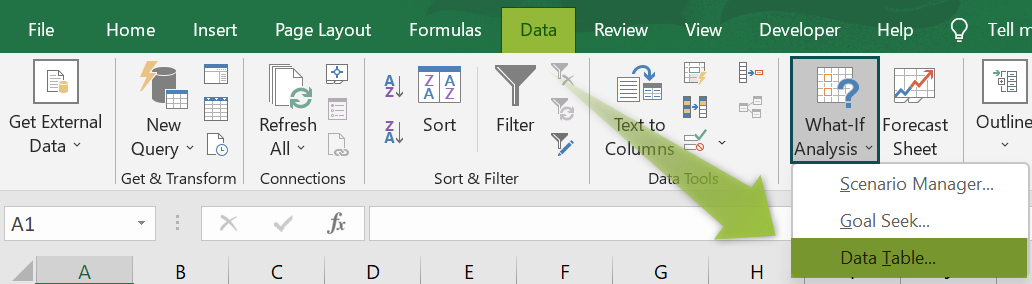2. Can we develop a row-oriented One-Variable Data Table in Excel?

We can develop a row-oriented One-Variable Data Table. Let us see the steps with an example.

The first table in the below image contains the parameters to solve a line equation for y-coordinates. Here, cell C6 shows the y-coordinate for the given parameter values in cells C3:C5. Also, the given single input variable is the x-coordinate, with its values in the row range F2:K2 in the second table.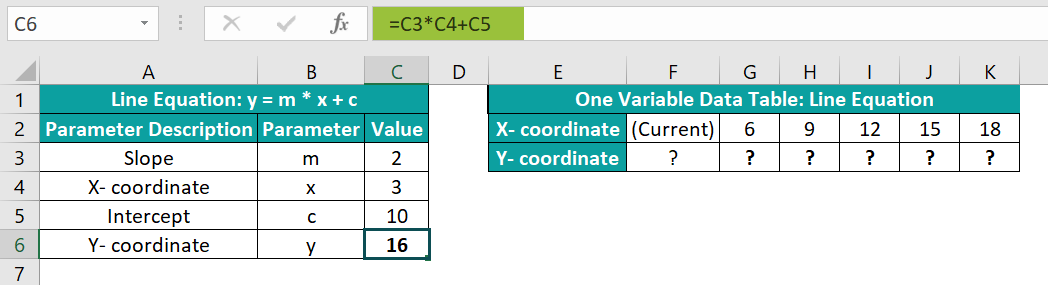The steps to create a Row-Oriented One-Variable Data Table are as follows:

• 1: Select cell F3 and provide a cell reference to the C6 cell’s formula.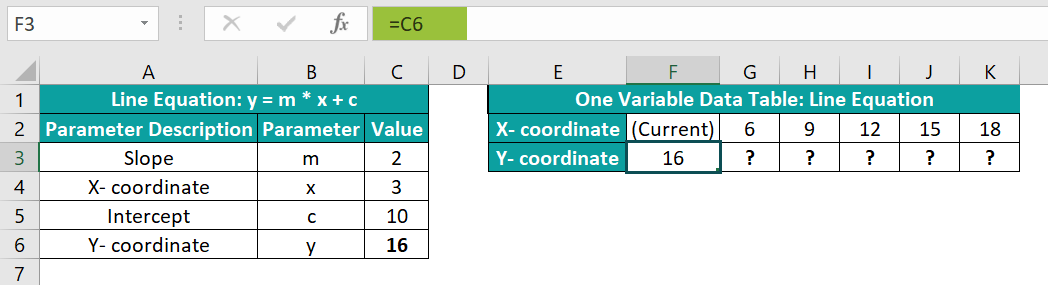• 2: Choose cell range, F2:K3 → select the “Data” tab → go to the “Forecast” group → click the “What-If Analysis” option drop-down → select the “Data Table” option, as shown below.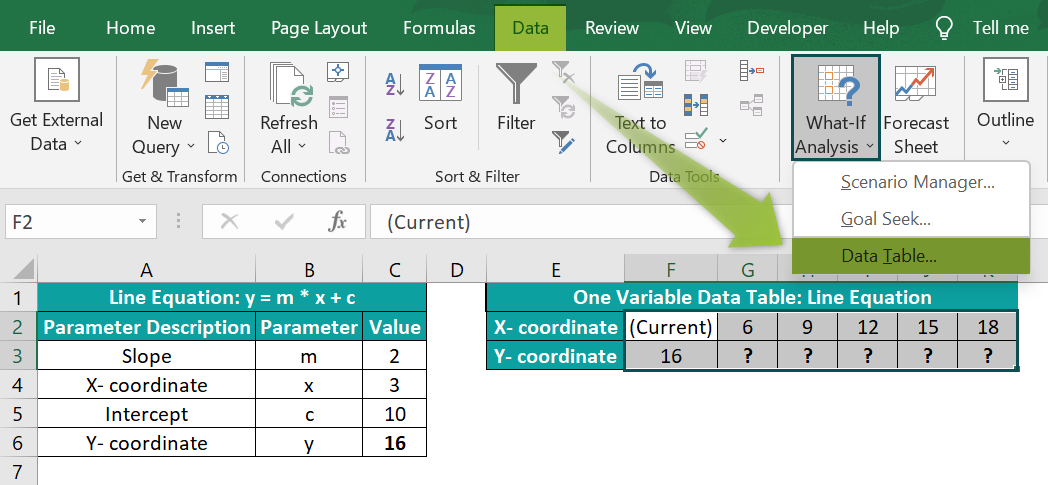• 3: In the “Data Table” dialog box that pops up, enter the cell reference to cell C4 in the “Row input cell:” field, as the given x-coordinate values are in a row range, as shown below.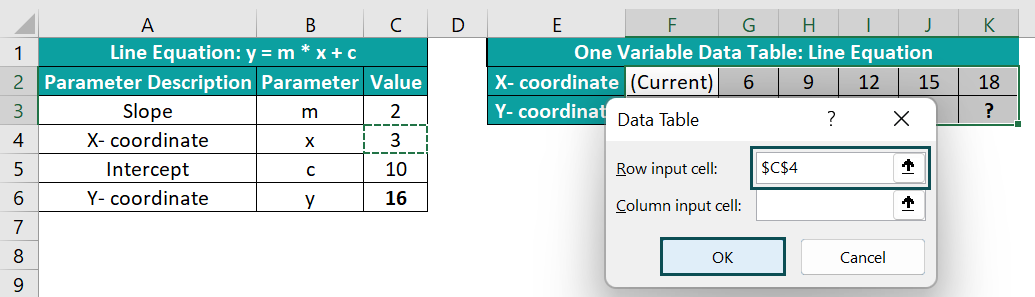• 4: Click “OK” in the Data Table window.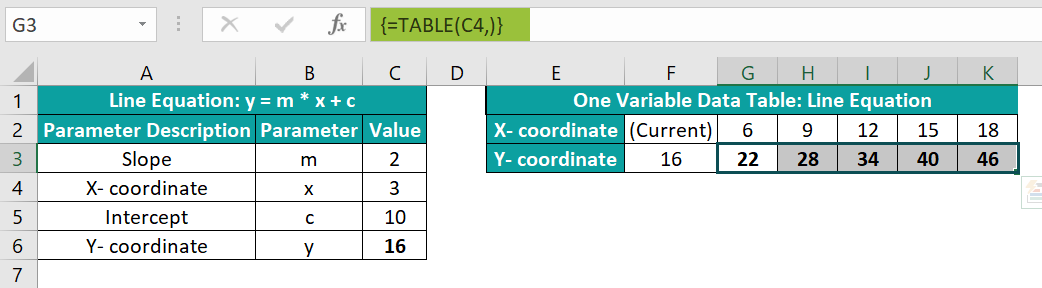We get the Row-Oriented One-Variable Data Table, as shown above.

3. Why is the one-variable data table not working in Excel?

The One-Variable Data Table may not work due to the following reasons:
We are trying to work on locked cells.
We incorrectly defined the input variable values. For example, the single input variable values are in a row. But we provide the column input cell reference in the Data Table dialog box while making a one-variable data table.